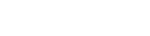# Optimizing Loops

The original code that I wrote to extract loops from the circuit node mesh does not necessarily obtain the smallest loops known as Windows. It traverses the mesh according to which nodes were placed first. So it tends to get a big overall loop followed by inner loops.

But, for circuit analysis, we want to have a set of the smallest loops. This is more efficient, and the standard way to do it. It’s easy to see these loops but harder to extract them in code.

I couldn’t think of a way to modify my original code, so the idea is to take the loops that it extracted and then process these. So after much head scratching and doodling, I came up with an algorithm to extract independent mesh windows:

• Compare 2 loops
• Find a common sequence of 3 or more nodes (cs)
• Designate the loops A and B where B is the shortest loop
• Replace inner nodes of cs in A with reversed B nodes minus cs nodes

And here is the code for it:

``````func minimize_loops(loops_):
var idxa = 0
while idxa < loops_.size() - 1:
var idxb = idxa + 1
# Look for subsequence of B in A
var a1 = -1
var b1 = -1
for bi in loops_[idxb].size():
var i = loops_[idxa].find(loops_[idxb][bi])
if i > -1:
a1 = i
b1 = bi
break
if b1 > -1:
var ai = a1
var bi = b1
var a2
var b2
var run_length = 1
# Scan in clockwise direction
while true:
ai += 1
# Wrap ai
if ai == loops_[idxa].size():
ai = 0
bi += 1
# Wrap bi
if bi == loops_[idxb].size():
bi = 0
if loops_[idxa][ai] != loops_[idxb][bi]:
break
a2 = ai
b2 = bi
run_length += 1
if run_length > 2:
# Got a subsequence
if loops_[idxa].size() > loops_[idxb].size():
var l = shrink_loop(idxa, idxb, a1, a2, b1, b2, loops_, true)
loops_[idxa] = loops_[idxb]
loops_[idxb] = l
else:
loops_[idxb] = shrink_loop(idxb, idxa, b1, b2, a1, a2, loops_, true)
else:
# Scan in anticlockwise direction
ai = a1
bi = b1
run_length = 1
while true:
ai -= 1
# Wrap ai
if ai < 0:
ai = loops_[idxa].size()
bi += 1
# Wrap bi
if bi == loops_[idxb].size():
bi = 0
if loops_[idxa][ai] != loops_[idxb][bi]:
break
a2 = ai
b2 = bi
run_length += 1
if run_length > 2:
# Got a subsequence
if loops_[idxa].size() > loops_[idxb].size():
var l = shrink_loop(idxa, idxb, a1, a2, b1, b2, loops_, false)
loops_[idxa] = loops_[idxb]
loops_[idxb] = l
else:
loops_[idxb] = shrink_loop(idxb, idxa, b1, b2, a1, a2, loops_, false)
idxa += 1

func shrink_loop(idxa, idxb, a1, a2, b1, b2, loops_, reverse):
var b_nodes = []
if b1 > 0:
b_nodes.append_array(loops_[idxb].slice(0, b1 - 1))
if b2 < loops_[idxb].size() - 1:
b_nodes.append_array(loops_[idxb].slice(b2 + 1, -1))
if reverse:
b_nodes.invert()
var loopa
if a2 > a1:
if a1 > 0:
loopa = loops_[idxa].slice(0, a1 - 1)
loopa.append_array(b_nodes)
if a2 < loops_[idxa].size() - 1:
loopa = loops_[idxa].slice(a2 + 1, -1)
else:
if a2 > 0:
loopa = loops_[idxa].slice(0, a2 - 1)
loopa.append_array(b_nodes)
if a1 < loops_[idxa].size() - 1:
loopa.append_array(loops_[idxa].slice(a1 + 1, -1))
return loopa
``````

In the above code, the array indices may loop around. Also, the smaller loop is put into the place of array A and the larger loop is put into the place of array B, so it is used as array A on the next iteration of testing for an inner loop.

This code in a scripting language may seem like it may be slow, but it only runs once to compile the data that we need for simulation of our circuit.

## More Devlog entries

Most recent first